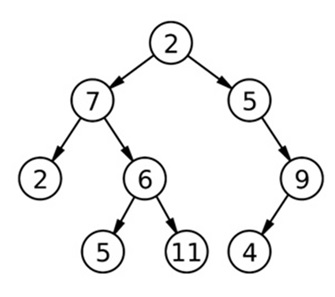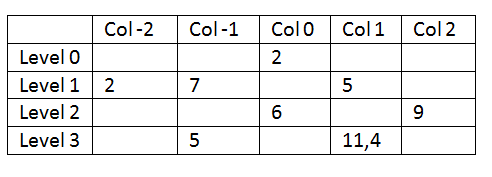Q:

# Bottom View of Binary Tree

Given a binary tree, print the bottom view from left to right.Example:

```    In the above example the bottom view is:
2 5 6 11 4 9 (left to right)```

What is bottom view?

Bottom view is not only the leaf nodes. We can consider the tree like below: (same as the problem of finding vertical sum)

For the above tree, let's check what the vertical sum is for the tree,Just consider, we have partitioned the tree nodes into column & rows where rows are the level no starting from 0. Then the above tree can be converted into the above table easily.

1. Start from root. Root column is 0.
2. If next node is at left of current node
Then column of next node = column of current node-1
Else
Column of next node = column of current node+1

Using the above steps the tree can be easily partitioned to the table. It is to observe that there may be several entries at a specific (row, column) position. Like here, at Level3, column no 1 has two entry 11 & 4.

Rest is about printing the last entry (bottommost) in each column. If there are multiple entries in the bottommost (in this case 11 & 4 for col 1) the last one entry is taken only.

Thus the output should be 2, 5, 6, 4, 9 (from col -2 to col 2 direction).

Though the visual description seems to be very easy to solve this problem, in programming view it's not that easy.

Algorithm to find vertical sum

The basic concept is to do pre-order traversal & while traversing we will keep track for each column (hashing) & make the entry.

Thus the column no is used as key & we need a map to process our algorithm.

1. Initialize map<int, int>hash. //(ordered map)
2. Initialize map<int, int>hash. //(ordered map)
Call findbottom(root, 0, hash);
3. Recursive function
```Function findbottom( tree node, column, reference of map hash)
a.  If (node== NULL)
Return;
//hash[column].push_back(node->data)
b.  Add node value in the column list;
// findbottom(node->left, column-1, reference of map hash)
c.  Recursive do for the left subtree;
We are passing col-1 since it's on the left
// findbottom(node->right, column+1, reference of map hash)
d.  Recursive do for the right subtree;
We are passing col+1 since it's on the right
End Function
```
4. Print the last entry only for each column to output the bottom view.

C++ implementation

``````#include <bits/stdc++.h>
using namespace std;

class tree{
public:
int data;
tree *left;
tree *right;
};

void findbottom(tree* root,map<int,vector<int>>& m, int col){
if(!root)
return;
m[col].push_back(root->data);
findbottom(root->left,m,col-1);
findbottom(root->right,m,col+1);
}

void bottomView(tree *root)
{
map<int,vector<int>> m;
//m.push_back(root->data);
findbottom(root,m,0);
for(auto it=m.begin();it!=m.end();it++){
cout<<(it->second)[(it->second).size()-1]<<" ";
}
}

// creating new node
tree* newnode(int data)
{
tree* node = (tree*)malloc(sizeof(tree));
node->data = data;
node->left = NULL;
node->right = NULL;
return(node);
}

int main()
{
//**same tree is builted as shown in example**
cout<<"Tree is built like the example aforesaid"<<endl;

//building the tree like as in the example
tree *root=newnode(2);
root->left= newnode(7);
root->right= newnode(5);
root->right->right=newnode(9);
root->right->right->left=newnode(4);
root->left->left=newnode(2);
root->left->right=newnode(6);
root->left->right->left=newnode(5);
root->left->right->right=newnode(11);

cout<<"printing bottom view......"<<endl;
bottomView(root);

return 0;
}
``````

Output

```Tree is built like the example aforesaid
printing bottom view......
2 5 6 4 9```